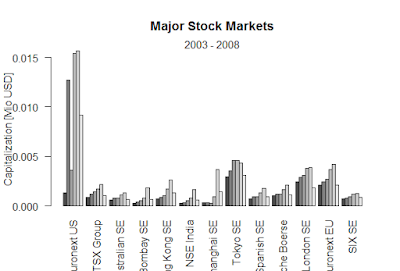Showing posts with label Lorenz model describes the dynamics of three state variables. Show all posts
Showing posts with label Lorenz model describes the dynamics of three state variables. Show all posts

## Lorenz model describes the dynamics of three state variables, X, Y and Z

> Lorenz <- function (t, y, parms) {
+     with(as.list(c(y, parms)), {
+         dX <- a * X + Y * Z
+         dY <- b * (Y - Z)
+         dZ <- -X * Y + c * Y - Z
+         return(list(c(dX, dY, dZ)))
+     })
+ }
> parms <- c(a = -8/3, b = -10, c = 28)
> yini <- c(X = 1, Y = 1, Z = 1)
> times <- seq(from = 0, to = 100, by = 0.01)
> out <- ode(y = yini, times = times, func = Lorenz, parms = parms)
>
> plot(out, lwd = 2)
> plot(out[,"X"], out[,"Y"],
+      type = "l", xlab = "X",
+      ylab = "Y", main = "butterfly")

### Black-Scholes formula-R

Black-Scholes formula-R > BlackScholes <- function(TypeFlag = c("c", "p"), S, X, Time, r, b, sigma) { TypeFla...